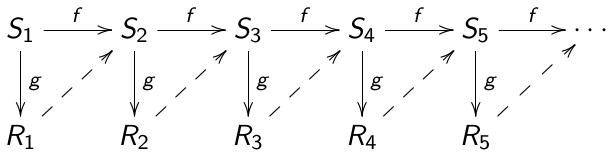# Dual EC DRBG

## Background on DRBGs and back doors

DRBG stands for "Deterministic Random Bit Generator", i.e. a deterministic method of deriving bits used as if they were random bits. More commonly such mechanisms are referred to as PRNGs, which stands for "Pseudo-Random Number Generators". "Pseudo-random" means that the outputs appear to be random even though they are not actually random, and saying "number" rather than "bit" reflects the fact that a typical PRNG produces many bits of output at once.

Here is a schematic illustration of how a typical PRNG works:At every step the inner state Si is updated, using the update function f to produce Si+1. At the same time the function g produces the output Ri, i.e. Si+1= f(Si) and Ri= g(Si).

The RNG is not secure if there is a way to obtain information on Si or Si+1 given Ri (or a sequence of several output values), as shown in the following illustration:In this case, the attacker has an advantage of reconstructing the inner state. Once Sj for some j is computed, the attacker can compute all further inner states and all further outputs. A hidden method of computing these diagonals is a back door.